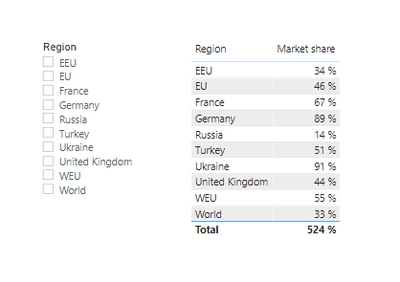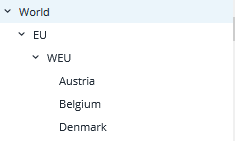cancel
Showing results for
Did you mean:

Fabric is Generally Available. Browse Fabric Presentations. Work towards your Fabric certification with the Cloud Skills Challenge.Helper I

## create right hierarchy filter for Continent, Region, Country

I have the following data fou our use case:We have market shares for countries as well as consolidated regions. Our "pain" is that we have all the data in the same column

We would like to create a hierarchy that is structured as follows:

World = World
EU = France, Germany, Russia, Turkey, Ukraine, United Kingdom
WEU = France, Germany, United Kingdom
EEU = Turkey, Ukraine, Russia

the final result in Power BI (Filter) should look like thisAt the end, the market shares should not be aggregated when filtering, but should refer to the market share provided in the column.

I would be grateful for any help!

1 ACCEPTED SOLUTIONCommunity Support

Hi @ys034 ,

I suggest you to create a calculated table for hierarchy level.

``````Table 2 =
SELECTCOLUMNS (
FILTER (
VALUES ( 'Table'[Region] ),
NOT ( [Region] IN { "World", "EU", "WEU", "EEU" } )
),
"Level1", "World",
"Level2", "EU",
"Level3",
IF (
'Table'[Region] IN { "France", "Germany", "United Kingdom" },
"WEU",
"EEU"
),
"Level4",'Table'[Region]
)``````

Table2 looks like as below.Then create a meause.

``````Measure =
VAR _LEVEL1 = CALCULATE(SUM('Table'[Market Share]),FILTER(ALL('Table'),'Table'[Region] = MAX('Table 2'[Level1])))
VAR _LEVEL2= CALCULATE(SUM('Table'[Market Share]),FILTER(ALL('Table'),'Table'[Region] = MAX('Table 2'[Level2])))
VAR _LEVEL3= CALCULATE(SUM('Table'[Market Share]),FILTER(ALL('Table'),'Table'[Region] = MAX('Table 2'[Level3])))
VAR _LEVEL4 =CALCULATE(SUM('Table'[Market Share]),FILTER(ALL('Table'),'Table'[Region] = MAX('Table 2'[Level4])))
RETURN
IF(ISINSCOPE('Table 2'[Level4]),_LEVEL4,IF(ISINSCOPE('Table 2'[Level3]),_LEVEL3,IF(ISINSCOPE('Table 2'[Level2]),_LEVEL2,IF(ISINSCOPE('Table 2'[Level1]),_LEVEL1,SUM('Table'[Market Share])))))``````

Result is as below.Best Regards,
Rico Zhou

If this post helps, then please consider Accept it as the solution to help the other members find it more quickly.Community Support

Hi @ys034 ,

I suggest you to create a calculated table for hierarchy level.

``````Table 2 =
SELECTCOLUMNS (
FILTER (
VALUES ( 'Table'[Region] ),
NOT ( [Region] IN { "World", "EU", "WEU", "EEU" } )
),
"Level1", "World",
"Level2", "EU",
"Level3",
IF (
'Table'[Region] IN { "France", "Germany", "United Kingdom" },
"WEU",
"EEU"
),
"Level4",'Table'[Region]
)``````

Table2 looks like as below.Then create a meause.

``````Measure =
VAR _LEVEL1 = CALCULATE(SUM('Table'[Market Share]),FILTER(ALL('Table'),'Table'[Region] = MAX('Table 2'[Level1])))
VAR _LEVEL2= CALCULATE(SUM('Table'[Market Share]),FILTER(ALL('Table'),'Table'[Region] = MAX('Table 2'[Level2])))
VAR _LEVEL3= CALCULATE(SUM('Table'[Market Share]),FILTER(ALL('Table'),'Table'[Region] = MAX('Table 2'[Level3])))
VAR _LEVEL4 =CALCULATE(SUM('Table'[Market Share]),FILTER(ALL('Table'),'Table'[Region] = MAX('Table 2'[Level4])))
RETURN
IF(ISINSCOPE('Table 2'[Level4]),_LEVEL4,IF(ISINSCOPE('Table 2'[Level3]),_LEVEL3,IF(ISINSCOPE('Table 2'[Level2]),_LEVEL2,IF(ISINSCOPE('Table 2'[Level1]),_LEVEL1,SUM('Table'[Market Share])))))``````

Result is as below.Best Regards,
Rico Zhou

If this post helps, then please consider Accept it as the solution to help the other members find it more quickly.Announcements#### Power BI Monthly Update - November 2023

Check out the November 2023 Power BI update to learn about new features.#### Fabric Community News unified experience

Read the latest Fabric Community announcements, including updates on Power BI, Synapse, Data Factory and Data Activator.#### Exclusive opportunity for Women!

Join us for a free, hands-on Microsoft workshop led by women trainers for women where you will learn how to build a Dashboard in a Day!#### The largest Power BI and Fabric virtual conference

130+ sessions, 130+ speakers, Product managers, MVPs, and experts. All about Power BI and Fabric. Attend online or watch the recordings.Top Solution Authors
Top Kudoed Authors
Users online (3,975)